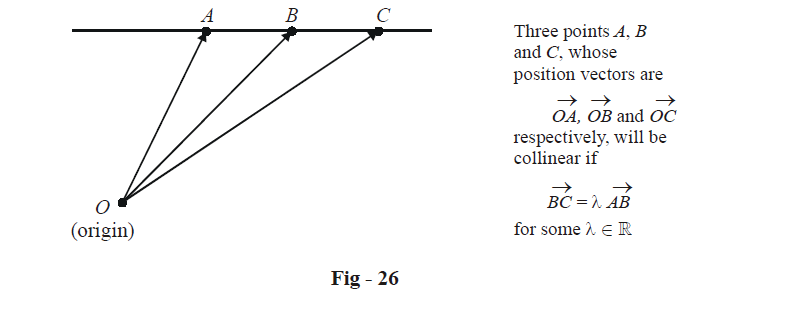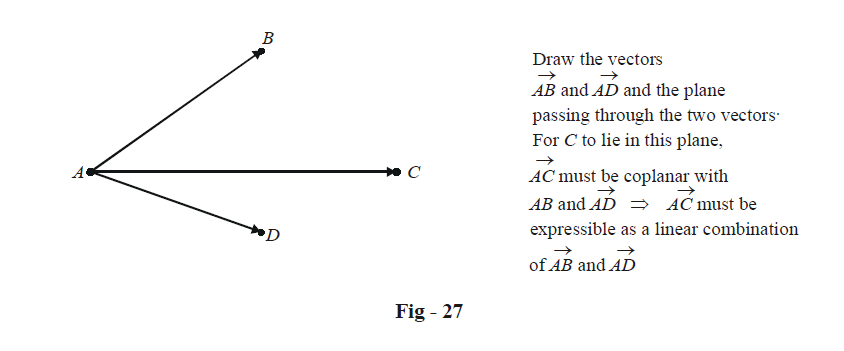# Examples On Basics Of Vectors Set-1

Go back to  'Vectors and 3-D Geometry'

Learn from the best math teachers and top your exams

• Live one on one classroom and doubt clearing
• Practice worksheets in and after class for conceptual clarity
• Personalized curriculum to keep up with school

Example – 6

Show that the vectors $$\hat i - 3\hat j + 2\hat k,\,\,2\hat i - 4\hat k - 4\hat k\,and\,\,3\hat i + 2\hat j - \hat k{\text{ }}$$ are linearly independent.

Solution: Let  $$\lambda ,\mu ,\gamma \in \mathbb{R}$$ be scalars such that

\begin{align}& \lambda \left( {\hat i - 3\hat j + 2\hat k} \right) + \mu \left( {2\hat i - 4\hat j - 4\hat k} \right) + \gamma \left( {3\hat i + 2\hat j - \hat k} \right) = \vec 0 \hfill \\\\ \Rightarrow \quad &\left( {\lambda + 2\mu + 3\gamma } \right)\hat i + \left( { - 3\lambda - 4\mu + 2\gamma } \right)\hat j + \left( {2\lambda - 4\mu - \gamma } \right)\hat k = \vec 0 \\\\ \Rightarrow \;\;\;\;& \quad \left. \begin{gathered}\,\,\,\,\,\,\lambda + 2\mu + 3\gamma = 0 \hfill \\\\ - 3\lambda - 4\mu + 2\gamma = 0 \hfill \\\\ \,\,\,\,\,2\lambda - 4\mu - \gamma = 0 \hfill \\\\ \end{gathered} \right\}\quad\quad\quad...\left( 1 \right)\end{align}

The determined of the coefficient matrix is

\left| {\begin{align}&\quad 1&2&&3 \\ &\;{ - 3}&{ - 4}&&2 \\&\quad 2&{ - 4}&&{ - 1}\end{align}} \right| \ne 0

Thus, the system of equations in(1) has no solution for $$\lambda ,\mu \,\,{\text{and}}\,\,\gamma$$apart from the trivial solution $$\lambda = \mu \,\, = \gamma = 0.$$ This implies that the three vectors are linearly independent.

Example – 7    COLLINEARITY OF POINTS

Let $$\vec a,\,\,\vec b\,\,{\text{and}}\,\vec c$$ be three non-coplanar vectors. Prove that the points $$A\left( {2\vec a + \vec b - \vec c} \right),\,\,B\left( {5\vec a - \vec b + 2c} \right)\,and\,C\left( {8\vec a - 3\vec b + 5\vec c} \right)$$ are collinear. When we say the point $$P\left( {\vec p} \right)$$ , we mean the point whose position vector, i.e, the vector drawn from the origin O  to that point,$$\overrightarrow {OP} ,$$ is $$\vec p$$ .

Solution: We have been given the position vectors of three points and we are required to prove that they are collinear. Let us see what condition must be satisfied in order for three points to be collinear:Thus, there must be some $$\lambda \in \mathbb{R}$$ for which

\begin{align}&\qquad\;\overrightarrow {BC} = \lambda \overrightarrow {AB} \hfill \\\\ & \Rightarrow \quad \overrightarrow {OC} - \overrightarrow {OB} = \lambda \left( {\overrightarrow {OB} - \overrightarrow {OA} } \right) \hfill \\\\&\Rightarrow \quad \lambda \overrightarrow {OA} - \left( {1 + \lambda } \right)\overrightarrow {OB} + \overrightarrow {OC} = \vec 0 \hfill \\\\& \Rightarrow \quad \lambda \left( {2\vec a + \vec b - \vec c} \right) - \left( {1 + \lambda } \right)\left( {5\vec a - \vec b + 2\vec c} \right) + \left( {8\vec a - 3\vec b + 5\vec c} \right) = \vec 0 \hfill \\\\&\Rightarrow \quad \left( {3 - 3\lambda } \right)\vec a + \left( {2\lambda - 2} \right)\vec b + \left( {3 - 3\lambda } \right)\vec c = \vec 0 \hfill \\ \end{align}

Since $$\vec a,\,\,\vec b\,\,{\text{and}}\,\vec c$$ are non-coplanar, we have

\begin{align}&3 - 3\lambda = 0 \hfill \\\\&2\lambda - 2 = 0 \hfill \\\\& 3 - 3\lambda = 0 \hfill \\ \end{align}

This consistently gives the solution $$\lambda = 1$$ , implying A, B and C are collinear.

Example – 8

Let $$\vec a,\,\,\vec b\,\,{\text{and}}\,\vec c$$ be three non-coplanar vectors. Prove that the points  $$A\left( {2\vec a + 3\vec b - \vec c} \right),\,B\left( {\vec a - 2\vec b - 3\vec c} \right),\,C\left( {3\vec a + 4\vec b - 2\vec c} \right)\,and\,D\left( {\vec a - 6\vec b + 6\vec c} \right)$$ are coplanar.

Solution: As in the previous example, we first draw a visual picture to determine when four points can be coplanar.Thus, as explained in the figure, we must have some scalars $$\lambda ,\mu \in \mathbb{R}$$ for which

\begin{align}& \qquad\quad\overrightarrow {AC} = \lambda \overrightarrow {AB} + \mu \overrightarrow {AD} \hfill \\\\&\Rightarrow \quad \left( {\overrightarrow {OC} - \overrightarrow {OA} } \right) = \lambda \left( {\overrightarrow {OB} - \overrightarrow {OA} } \right) + \mu \left( {\overrightarrow {OD} - \overrightarrow {OA} } \right)\left\{ {O\;is{\text{ }}the{\text{ }}origin} \right\} \hfill \\\\ & \Rightarrow \quad \vec a + \vec b - \vec c = \lambda \left( { - \vec a - 5\vec b + 4\vec c} \right) + \mu \left( { - \vec a - 9\vec b + 7\vec c} \right) \hfill \\\\ &\Rightarrow \quad \left( {1 + \lambda + \mu } \right)\vec a + \left( {1 + 5\lambda + 9\mu } \right)\vec b + \left( { - 1 - 4\lambda - 7\mu } \right)\vec c = \vec 0 \hfill \\ \end{align}

Since $$\vec a,\,\,\vec b\,\,{\text{and}}\,\vec c$$ are non-coplanar, we must have

\begin{align}&1 + \;\lambda + \; \mu\; = 0 \hfill \\\\& 1 + 5\lambda + 9\mu = 0 \hfill \\\\&1 + 4\lambda + 7\mu = 0 \hfill \\ \end{align}

As can be easily verified, this system has the solution $$\lambda = - 2,\mu = 1,$$ implying $$\overrightarrow {AB} ,\,\,\overrightarrow {AC} \,\,{\text{and}}\,\,\overrightarrow {AD}$$ are indeed coplanar.

Thus, the points A, B, C and D are coplanar.

Vectors
grade 11 | Questions Set 1
Vectors
Vectors
grade 11 | Questions Set 2
Vectors
Three Dimensional Geometry
grade 11 | Questions Set 2
Three Dimensional Geometry
Three Dimensional Geometry
grade 11 | Questions Set 1
Three Dimensional Geometry
Vectors
grade 11 | Questions Set 1
Vectors
Vectors
grade 11 | Questions Set 2
Vectors